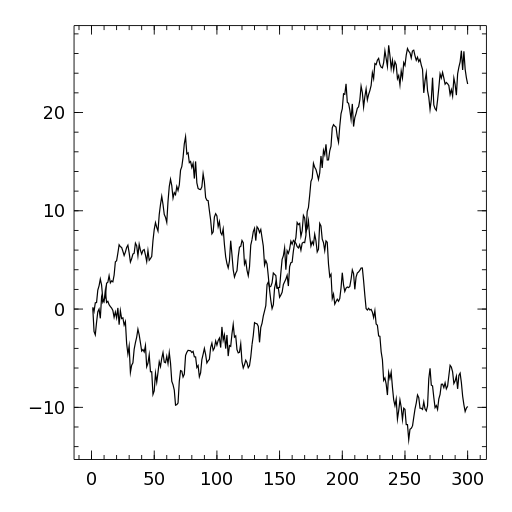# oplot¶

oplot(...)

Takes the same arguments as plot(), but overplots the current plot.

## Example¶

randomwalk(n) = (r = randn(n); [sum(r[1:i]) for i=1:n])
plot()
oplot(randomwalk(300))
oplot(randomwalk(300))